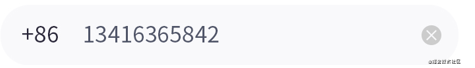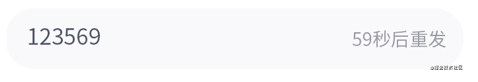# vue3如何優雅的實現移動端登錄註冊模塊

## 前言## 輸入框組件### 佈局

```<template>
<div class="input">
<!--左側,lt是左側內容-->
<span class="left-text">
{{ lt }}
</span>

<!--中間-->
<input class="content" v-bind="\$attrs" :value="value" @input="onChange" />

<!--右側,rt判端是驗證碼還是叉叉圖標-->
<div v-if="rt == 'timer'" class="right-section">
{{ timerData.content }} <!--可能是'驗證碼',也可能是倒計時 -->
</div>
<div
v-else-if="rt == 'close'"
class="right-section"
>
<van-icon name="close" /> <!--叉叉圖標-->
</div>
</div>
</template>
```

Demo 效果圖如下:### v-model

``` <InputForm
lt="+86" <!--左側顯示+86-->
rt="close" <!--右側顯示叉叉圖標-->
placeholder="請輸入手機號碼"
/>
```

``` const form_data = reactive({
number_number: '', //用戶名
password: '', //密碼
ppassword: '', //重復密碼
captcha: '', //驗證碼
})
```

``` <InputForm
lt="+86"  <!--左側顯示+86-->
rt="close" <!--右側顯示叉叉圖標-->
placeholder="請輸入手機號碼"
v-model:value="form_data.password"
/>
```

```<template>
<div class="input">
...
<input class="content" v-bind="\$attrs" :value="value" @input="onChange" />
...
</div>
</template>
export default defineComponent({
props: {
lt:String,
rt: String,
value: String
},
setup(props, context) {
const onChange = (e:KeyboardEvent) => {
const value = (e.target as HTMLInputElement).value;
context.emit("update:value",value);
};
return {
onChange
}
}
})
```

oninput事件 的回調函數將獲取到的值使用 context.emit(“update:value”,value) 返回回去.

## 數據校驗

``` <Form ref="form" :rules="rules">

<InputForm
lt="+86"
rt="close"
v-model:value="form_data.number_number"
placeholder="請輸入手機號碼"
propName="number_number"
/>

<InputForm
rt="timer"
v-model:value="form_data.captcha"
placeholder="請輸入手機驗證碼"
propName="captcha"
/>

<InputForm
rt="close"
v-model:value="form_data.password"
placeholder="請輸入密碼"
type="password"
propName="password"
/>

<InputForm
rt="close"
v-model:value="form_data.ppassword"
placeholder="請輸入確認密碼"
type="password"
propName="ppassword"
/>

<Button text="註 冊" @sub="onSubmmit" /> <!--註冊按鈕-->

</Form>
```

```export default defineComponent({
components: {
InputForm, //輸入框
Button, //註冊按鈕
Form, //Form組件
},
setup(props) {

const form_data = ...; //省略

const rules = ...;

//獲取最外層Form組件的實例
const form = ref(null);

const onSubmmit = ()=>{
if (!form.value || !form.value.validate()) {
return false;
}
//校驗通過瞭,可以請求註冊接口瞭
}

return {
form,
rules,
onSubmmit,
form_data
};
},
});
```

### rules設計

rules 是一個對象,例如上述註冊頁面的 rules 定義如下:

```const rules = {
number_number:[{
type: 'required',
msg:"請輸入正確的手機號"
}
"phone"
],
captcha:[
{
type: 'required',
msg: '驗證碼不能為空'
}
],
password: [
{
type: 'required',
msg: '請輸入密碼',
},
{
type: 'minLength',
params: 6,
msg: '密碼長度不能小於6位',
},
],
ppassword:[
{
type: 'custome',
callback() {
if (form_data.password !== form_data.ppassword) {
return {
flag: false,
msg: '兩次輸入的密碼不一致',
};
}
return {
flag: true,
};
},
},
]
}
```

``` {
type, // 類型
msg, //自定義的錯誤信息
params, //傳過來的參數值 比如 {type:'minLength',params:6},值最小長度不能低於6位
callback //自定義校驗函數
}
```

type 是校驗類型,它如果填 required ,表示是必填項.如果用戶沒填,點擊註冊按鈕提交時就會報出 msg 定義的錯誤信息.

### Form表單

rules 被定義好後傳給 Form 組件, Form 組件需要將校驗邏輯分發給它的子組件.讓其每個子組件都負責生成自己的校驗函數.

```<!-- 表單組件 -->
<template>
<div class="form">
<slot></slot>
</div>
</template>

<script lang="ts">
import { ref, provide } from "vue";
export default defineComponent({
name: "Form",
props:{
rules:Object
},
setup(props) {

...//省略

provide("rules",props.rules); // 將校驗規則分發下去

const validate = ()=>{
//向外暴露的校驗函數
}

return {
validate
}
}
})
</script>
```

### 子組件生成校驗函數

```import { inject，onMounted } from "vue";
...

setup(props, context) {

const rules = inject("rules");

const rule = rules[props.propName];// 通過propName拿到校驗規則

const useValidate = () => {
const validateFn = getValidate(rule); // 獲取校驗函數
const execValidate = () => {
return validateFn(props.value); //執行校驗函數並返回校驗結果
};
onMounted(() => {
const Listener = inject('collectValidate');
if (Listener) {
Listener(execValidate);
}
});
};

useValidate(); //初始化校驗邏輯
...
}
```

rules 結構類似如下.通過 inject 和 propName 可以拿到 Form 分發給該輸入框要執行的規則 rule .

```{
captcha:[{
type: 'required',
msg: '驗證碼不能為空'
}],
password:[{
type: 'required',
msg: '請輸入密碼',
}]
}
```

```setup(props) {

const list = ref([]);//定義一個數組

const listener = (fn) => {
list.value.push(fn);
};

provide("collectValidate", listener); //將監聽函數分發下去

//驗證函數
const validate = (propName) => {
const array = list.value.map((fn) => {
return fn();
});
const one = array.find((item) => {
return item.flag === false;
});
if (one && one.msg) {
//驗證不通過
Alert(one.msg);//彈出錯誤提示
return false;
} else {
return true;
}
};
...

```

### 校驗

```class Validate {

constructor() {}

required(data) { //校驗是否為必填
const msg = '該信息為必填項'; //默認錯誤信息
if (data == null || (typeof data === 'string' && data.trim() === '')) {
return {
flag:false,
msg
}
}
return {
flag:true
}
}

//校驗是否為手機號
phone(data) {
const msg = '請填寫正確的手機號碼'; //默認錯誤信息
const flag = /^1\d{9}\$/.test(data);
return {
msg,
flag
}
}

//校驗數據的最小長度
minLength(data, { params }) {

let minLength = params; //最小為幾位

if (data == null) {
return {
flag:false,
msg:"數據不能為空"
}
}

if (data.trim().length >= minLength) {
return {flag:true};
} else {
return {
flag:false,
msg:`數據最小長度不能小於\${minLength}位`
}
}
}

}
```

Validate 類定義的所有方法中,第一個參數 data 是被校驗的值,第二個參數是在頁面定義每條 rule 中的規則.形如 {type: ‘minLength’, params: 6, msg: ‘密碼長度不能小於6位’} .

Validate 類中每個方法最終的返回的數據結構形如 {flag:true,msg:””} .結果中 flag 就來標識校驗是否通過, msg 為錯誤信息.

``` const getInstance = (function(){
let _instance;
return function(){
if(_instance == null){
_instance = new Validate();
}
return _instance;
}
})()
```

```/**
* 生成校驗函數
*/
export const getValidate = (rule) => {
const ob = getInstance();//獲取 Validate類 實例對象
const fn_list = []; //將所有的驗證函數收集起來
//遍歷rule數組,根據其類型獲取Validate類中的校驗方法放到fn_list中收集起來
rule.forEach((item) => {
if (typeof item === 'string') { // 字符串類型
fn_list.push({
fn: ob[item],
});
} else if (isRuleType(item)) { // 對象類型
fn_list.push({
//如果item.type為custome自定義類型,校驗函數直接使用callback.否則從ob實例獲取
...item,
fn: item.type === 'custome' ? item.callback : ob[item.type],
});
}
});
//需要返回的校驗函數
const execuate = (value) => {
let flag = true,
msg = '';
for (let i = 0; i < fn_list.length; i++) {
const item = fn_list[i];
const result = item.fn.apply(ob, [value, item]);//item.fn對應著Validate類定義的的校驗方法
if (!result.flag) {
//驗證沒有通過
flag = false;
msg = item.msg ? item.msg : result.msg;//是使用默認的報錯信息還是用戶自定義信息
break;
}
}
return {
flag,
msg,
};
};
return execuate;
};
```

rule 的數據結構形類似如下代碼.當把 rule 傳入 getValidate 函數,它會判端是對象還是字符串,隨後將其類型對應的校驗函數從 ob 實例中獲取存儲到 fn_list 中.

``` [
{
type: 'required',
msg: "請輸入電話號碼"
},
"phone"
]
```

getValidate 函數最終返回 execuate 函數,此函數也正是輸入框組件得到的校驗函數.在輸入框組件裡是可以拿到輸入框值的,如果將值傳給 execuate 方法調用.方法內部就會遍歷之前緩存的校驗函數列表 fn_list ,將值傳入每個校驗方法運行就能獲取該輸入框組件對當前值的校驗結果並返回回去.

## 最終效果